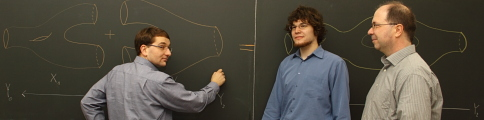# Research Interests

## Probability Theory

• (infinite dimensional) stochastic analysis
• Dirichlet forms
• Markov processes
• infinite dimensional diffusion processes
• random fields
• infinite dimensional stochastic differential equations
• martingale problems
• invariant measures
• ergodicity
• stochastic partial differential equations
• analysis on Wiener space
• measure valued diffusions

## Analysis

• coercive forms
• capacity theory
• operator theory, in particular, semigroups
• partial differential equations, in particular, elliptic and parabolic regularity
• functional inequalities

## Mathematical Physics

• mathematical statistical mechanics
• Euclidian quantum field theory